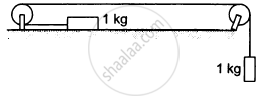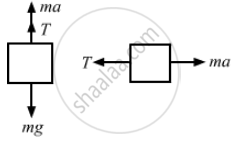Advertisement Remove all ads

# Calculate the Tension in the String Shown in Figure (5−E13). the Pulley and the String Are Light and All the Surfaces Are Frictionless. Take G = 10 M/S2. - Physics

Sum

Calculate the tension in the string shown in the following figure. The pulley and the string are light and all the surfaces are frictionless. Take g = 10 m/s2.Advertisement Remove all ads

#### Solution

The free-body diagrams for both the bodies are shown below:T + ma =mg    ...(i)
and T = ma    ...(ii)

From equations (i) and (ii), we get:
ma + ma = mg
⇒ 2ma = g
$\Rightarrow a = \frac{g}{2} = \frac{10}{5} = 5 m/ s^2$
From equation (ii),
T = ma = 5 N

Is there an error in this question or solution?
Advertisement Remove all ads

#### APPEARS IN

HC Verma Class 11, 12 Concepts of Physics 1
Chapter 5 Newton's Laws of Motion
Q 30 | Page 81
Advertisement Remove all ads
Advertisement Remove all ads
Share
Notifications

View all notifications

Forgot password?